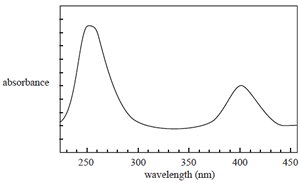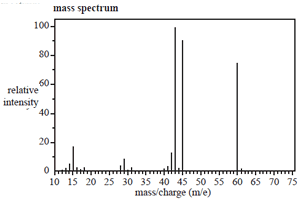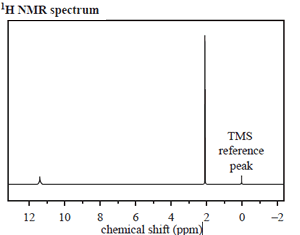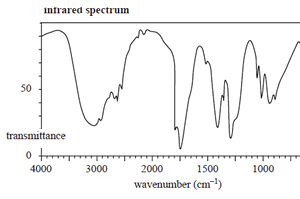Spectroscopy questions-2009 Question 1 The most appropriate technique to determine levels of the Pb2+ ion in blood is A. mass spectrometry. B. infrared spectroscopy. C. atomic absorption spectroscopy. D. high-performance liquid chromatography. Solution Question 2 A sample of compound M is analysed in a mass spectrometer where it forms the molecular ion M+ according to Answer C   Answer B Peaks on a mass spectrum are associated with ions – both parent molecular and fragment. These are the species deflected and separated in the magnetic field. M + e– → M+ + 2e- Some of the molecular ions fragment as follows. M+ → A+ + B and M+ → A + B+ The mass spectrum would show peaks due to the species A. M+, A, A+, B and B+ only. B. M+, A+ and B+ only. C. A+and B+ only. D. A and B only. Solution Question 3 The UV-visible spectrum of a solution of a certain compound is shown on the right. Consider the following statements about this compound and its UV-visible spectrum. I The amount of light absorbed by a solution of this compound depends on its concentration. II The amount of light absorbed by a solution of this compound depends on the wavelength of light used. III The spectrum is a result of electrons falling back from higher to lower electronic energy levels. IV The concentration of a solution of this compound can only be determined by UV-visible spectroscopy at 250 nm. Which of the above statements are true? A. I and II B. II and III C. I, II and III D. I, II and IV Solution Click to see the spectrum.Question 4 The structure of an organic molecule, with empirical formula CH2O, is determined using spectroscopic techniques. The mass spectrum, 1H NMR and infrared spectrumspectrum for this molecule are given on the right. Use the information provided by these spectra to answer the following questions. a. What is the molecular formula of this molecule? Solution Click to see the mass spectrumb. How many different proton environments are there in this molecule? Solution Click to see the 1H NMR spectrum.c. Draw the structure of the unknown molecule, clearly showing all bonds.   Solution Click to see the infrared spectrumd) Explain how the structure of the compound you have drawn in part c. is consistent with its IR spectrum. Solution Click to see the infrared spectrum e. Name the compound you have drawn in part c. Solution Click to see the infrared spectrum Continue with questions from the 2008 exam. Continue with general spectroscopy questions